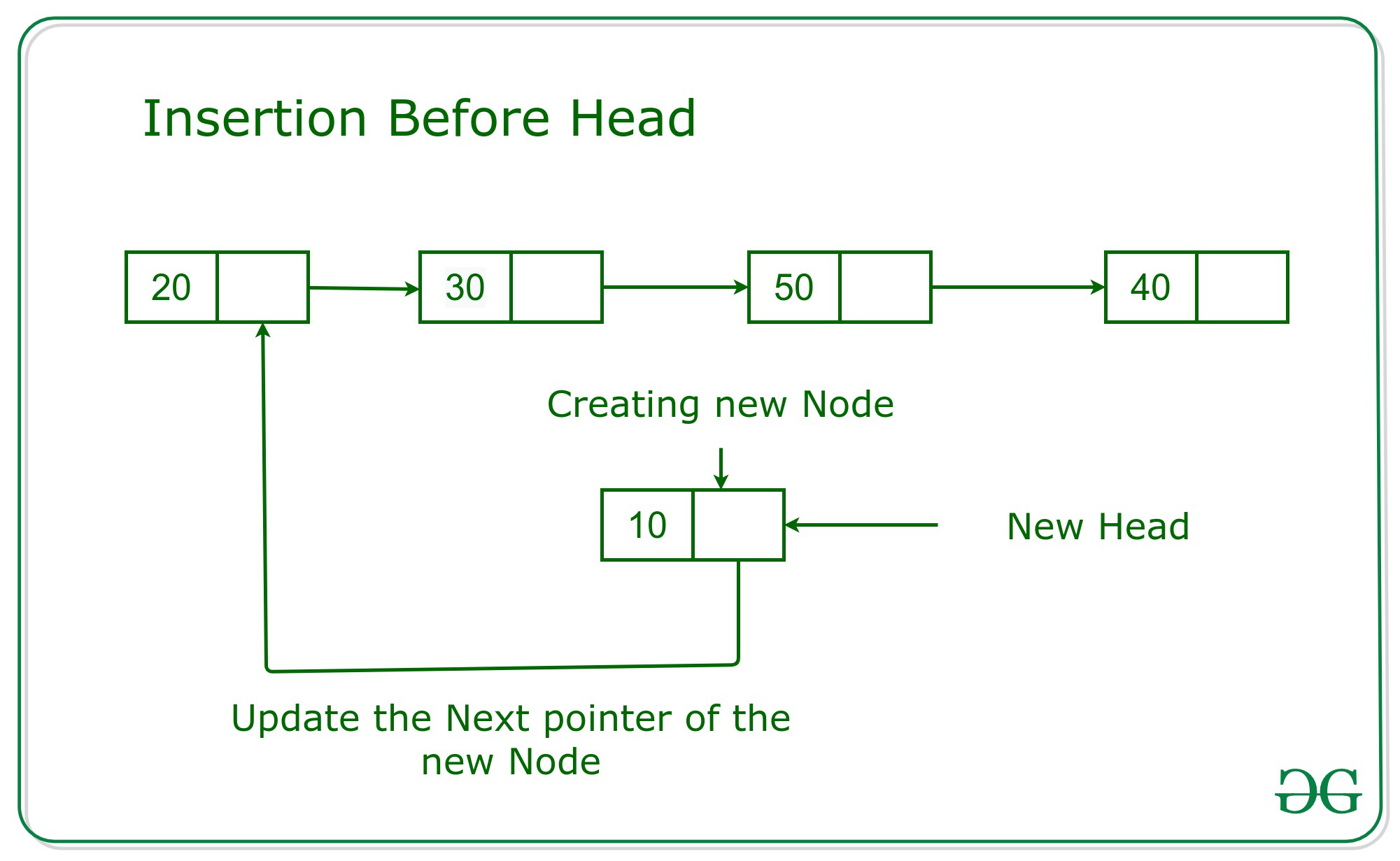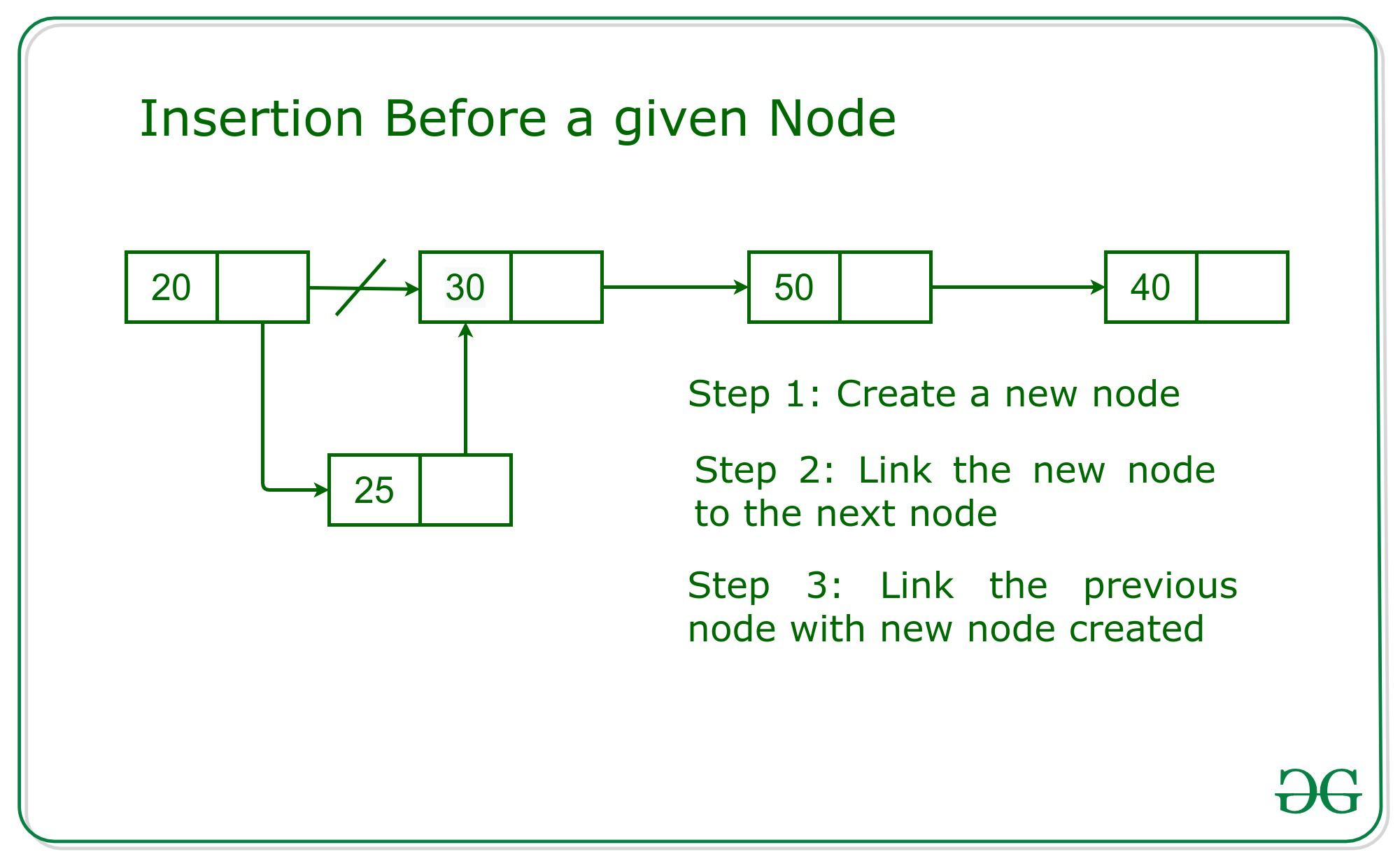Insert a node in Linked List before a given node

• Difficulty Level : Basic
• Last Updated : 24 Sep, 2021

Given a node of Linked List N and a value K, the task is to insert the node with value K in the linked list before the given node N.

Structure of the Node:

C++

 // Structure of Nodestruct Node {    int data;    Node* next;     // Constructor of Node    Node(int val, Node* link = 0)        : data(val), next(link)    {    }};

Java

 // Structure of Nodepublic class Node{    public int data;    public Node next;       // Constructor of Node    public Node(int val, Node link = null)    {        this.data = val;        this.next = link;    }} // This code is contributed by divyesh072019

Python3

 # Structure of Nodeclass Node:         # Constructor of Node    def __init__(self, val, link = None):                 self.data = val        self.next = link         # This code is contributed by pratham76

C#

 // Structure of Nodepublic class Node{    public int data;    public Node next;       // Constructor of Node    public Node(int val, Node link = null)    {        this.data = val;        this.next = link;    }}; // This code is contributed by rutvik_56

Javascript


Output:
5 8 6

In the given problem there might be two cases:

• Given node is the head node.
• Given node is any valid node except the head.

When given Node is the Head Node:

The idea is to create a new node with the given value K. Then the next part of the new node will be updated with the pointer head. And finally, the head will be updated with the new node’s address. Below is the image of the same:When given Node is any valid node except head node:

The simplest approach is to traverse the given linked list to search the previous node of the given node. Then, create the new node with the given value K.Now, update the next part of the new node with the address of the given node and the next part of the previous node with the address of the new node. Below is an illustration of the approach with the help of image:Below is the implementation of the above approach:

Javascript


Output:
5 8 6

Time Complexity: O(N)
Auxiliary Space: O(1)

My Personal Notes arrow_drop_up